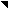# MINLPLib

### A Library of Mixed-Integer and Continuous Nonlinear Programming Instances

#### Removed Instance meanvar

 Formatsⓘ ams gms lp mod nl osil pip py Primal Bounds (infeas ≤ 1e-08)ⓘ 5.24339907 p1 ( gdx sol ) (infeas: 2e-17) Other points (infeas > 1e-08)ⓘ Dual Boundsⓘ 5.24339907 (ANTIGONE)5.24339907 (BARON)5.24339907 (COUENNE)5.24339907 (CPLEX)5.24339907 (LINDO)5.24339907 (SCIP) Referencesⓘ Dahl, H, Meeraus, Alexander, and Zenios, Stavros A, Some Financial Optimization Models: Risk Management. In Zenios, Stavros A, Ed, Financial Optimization, Cambridge University Press, New York, NY, 1993. Sourceⓘ GAMS Model Library model meanvar Applicationⓘ Financial Optimization Added to libraryⓘ 31 Jul 2001 Removed from libraryⓘ 16 Feb 2022 Removed becauseⓘ Instance is continuous and convex. Problem typeⓘ QP #Variablesⓘ 8 #Binary Variablesⓘ 0 #Integer Variablesⓘ 0 #Nonlinear Variablesⓘ 7 #Nonlinear Binary Variablesⓘ 0 #Nonlinear Integer Variablesⓘ 0 Objective Senseⓘ min Objective typeⓘ quadratic Objective curvatureⓘ convex #Nonzeros in Objectiveⓘ 7 #Nonlinear Nonzeros in Objectiveⓘ 7 #Constraintsⓘ 2 #Linear Constraintsⓘ 2 #Quadratic Constraintsⓘ 0 #Polynomial Constraintsⓘ 0 #Signomial Constraintsⓘ 0 #General Nonlinear Constraintsⓘ 0 Operands in Gen. Nonlin. Functionsⓘ Constraints curvatureⓘ linear #Nonzeros in Jacobianⓘ 15 #Nonlinear Nonzeros in Jacobianⓘ 0 #Nonzeros in (Upper-Left) Hessian of Lagrangianⓘ 49 #Nonzeros in Diagonal of Hessian of Lagrangianⓘ 7 #Blocks in Hessian of Lagrangianⓘ 1 Minimal blocksize in Hessian of Lagrangianⓘ 7 Maximal blocksize in Hessian of Lagrangianⓘ 7 Average blocksize in Hessian of Lagrangianⓘ 7.0 #Semicontinuitiesⓘ 0 #Nonlinear Semicontinuitiesⓘ 0 #SOS type 1ⓘ 0 #SOS type 2ⓘ 0 Minimal coefficientⓘ 5.0100e-02 Maximal coefficientⓘ 7.0890e+01 Infeasibility of initial pointⓘ 1 Sparsity JacobianⓘSparsity Hessian of Lagrangianⓘ```\$offlisting
*
*  Equation counts
*      Total        E        G        L        N        X        C        B
*          3        3        0        0        0        0        0        0
*
*  Variable counts
*                   x        b        i      s1s      s2s       sc       si
*      Total     cont   binary  integer     sos1     sos2    scont     sint
*          9        9        0        0        0        0        0        0
*  FX      1
*
*  Nonzero counts
*      Total    const       NL      DLL
*         23       16        7        0
*
*  Solve m using NLP minimizing objvar;

Variables  objvar,x2,x3,x4,x5,x6,x7,x8,x9;

Positive Variables  x3,x4,x5,x6,x7,x8,x9;

Equations  e1,e2,e3;

e1.. -0.5*(42.18*x3*x3 + 20.18*x3*x4 + 10.88*x3*x5 + 5.3*x3*x6 + 12.32*x3*x7 +
23.84*x3*x8 + 17.41*x3*x9 + 20.18*x4*x3 + 70.89*x4*x4 + 21.58*x4*x5 +
15.41*x4*x6 + 23.24*x4*x7 + 23.8*x4*x8 + 12.62*x4*x9 + 10.88*x5*x3 + 21.58
*x5*x4 + 25.51*x5*x5 + 9.6*x5*x6 + 22.63*x5*x7 + 13.22*x5*x8 + 4.7*x5*x9
+ 5.3*x6*x3 + 15.41*x6*x4 + 9.6*x6*x5 + 22.33*x6*x6 + 10.32*x6*x7 + 10.46
*x6*x8 + x6*x9 + 12.32*x7*x3 + 23.24*x7*x4 + 22.63*x7*x5 + 10.32*x7*x6 +
30.01*x7*x7 + 16.36*x7*x8 + 7.2*x7*x9 + 23.84*x8*x3 + 23.8*x8*x4 + 13.22*
x8*x5 + 10.46*x8*x6 + 16.36*x8*x7 + 42.23*x8*x8 + 9.9*x8*x9 + 17.41*x9*x3
+ 12.62*x9*x4 + 4.7*x9*x5 + x9*x6 + 7.2*x9*x7 + 9.9*x9*x8 + 16.42*x9*x9)
+ objvar =E= 0;

e2..    x2 - 0.1287*x3 - 0.1096*x4 - 0.0501*x5 - 0.1524*x6 - 0.0763*x7
- 0.1854*x8 - 0.062*x9 =E= 0;

e3..    x3 + x4 + x5 + x6 + x7 + x8 + x9 =E= 1;

* set non-default bounds
x2.fx = 0.115;
x3.up = 1;
x4.up = 1;
x5.up = 1;
x6.up = 1;
x7.up = 1;
x8.up = 1;
x9.up = 1;

Model m / all /;

m.limrow=0; m.limcol=0;
m.tolproj=0.0;

\$if NOT '%gams.u1%' == '' \$include '%gams.u1%'

\$if not set NLP \$set NLP NLP
Solve m using %NLP% minimizing objvar;

```

Last updated: 2023-08-16 Git hash: 2519540e